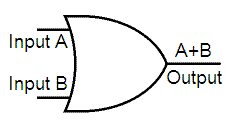# Logical OR - (logic OR gate)

A | B
performs a logical OR of arrays A and B and returns an array   containing elements set to either logical 1 (TRUE) or logical 0 (FALSE). Sometimes, this operation is considered an 'addition' in terms of electronic gates. This is the truth table and the representation of an OR Gate as it's seen in digital electronics.

 A    B    A | B 0    0      0 0    1      1 1    0      1 1    1      1This is a logical OR Gate, as used in electronic circuits

An element of the output array is set to 1 if either input array contains a non-zero element at that same array location. Otherwise, that element is set to 0.  A and B must have the same dimensions unless one is a scalar.  This is a simple example on how you can code it in Matlab.

Example 1:

If matrix A is:
A =

0     0     1     1
1     1     0     0
0     0     0     0
1     1     1     1

and matrix B is:
B =

0     0     0     0
1     1     1     1
0     1     0     1
1     0     1     0

Then, the OR operation between A and B is:

>> A | B

ans =

0     0     1     1
1     1     1     1
0     1     0     1
1     1     1     1

>>

Example 2:

If vector x is:
x =  0     1     2     3     0

and vector y is:
y =  1     2     3     0     0

Then, the OR operation between x and y is:

>> x | y

ans =  1     1     1     1     0

From 'Logical OR' to home

From 'Logical OR' to 'Boolean Algebra'

 Top Logical AND Logical XOR Logical NOT Boolean Axioms De Morgan's Laws